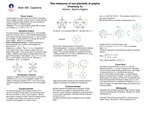## Stander Symposium Projects

#### Title

Two Measures of Non-Planarity of Graphs

Chucheng Yu

#### Description

This presentation is for a MTH 480 capstone project. We discuss the topic of planar graphs in the study of graph theory. A graph is a mathematical object consisting of two sets: a set of vertices and a set of edges, where an edge between two vertices depicts a relationship between those vertices. A planar graph can be drawn in the plane without edges crossing. For any given graph G, the crossing number is the minimum number of times edges in G cross each other, while the thickness number is the minimum number of planar subgraphs of G into which G can be decomposed. We will explore and compare these measures of non-planarity.

4-22-2021

#### Project Designation

Capstone Project

Aparna W. Higgins

Mathematics

#### Keywords

Stander Symposium Posters, College of Arts and SciencesCOinS